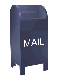The Inverse Discrete Fourier Transform (IDFT)

The Fourier transform takes a signal in the so called time domain (where each sample in the signal is associated with a time) and maps it, without loss of information, into the frequency domain. The frequency domain representation is exactly the same signal, in a different form. The inverse Fourier tranform maps the signal back from the frequency domain into the time domain.

A time domain signal will usually consist of a set of real values, where each value has an associated time (e.g., the signal consists of a time series). The Fourier transform maps the time series into a a frequency domain series, where each value is a complex number that is associated with a given frequency. The inverse Fourier transform takes the frequency series of complex values and maps them back into the original time series. Assuming that the original time series consisted of real values, the result of the IDFT will be complex numbers where the imaginary part is zero.

The Java code for the inverse Fourier tranform can be downloaded here

The perfect invertability of the Fourier transform is an important property for building filters which remove noise or particular components of a signals spectrum. Although frequency artifacts are common in the result of a Fourier transform, these artifacts do not result in error in the inverted signal. Wavelets are powerful tools for analyzing signals, but there can be errors when the result of a wavelet transform is inverted.

Ian Kaplan, September 2001
Revised: